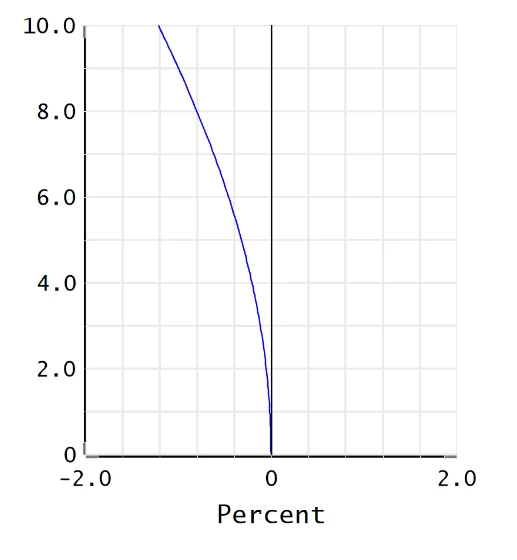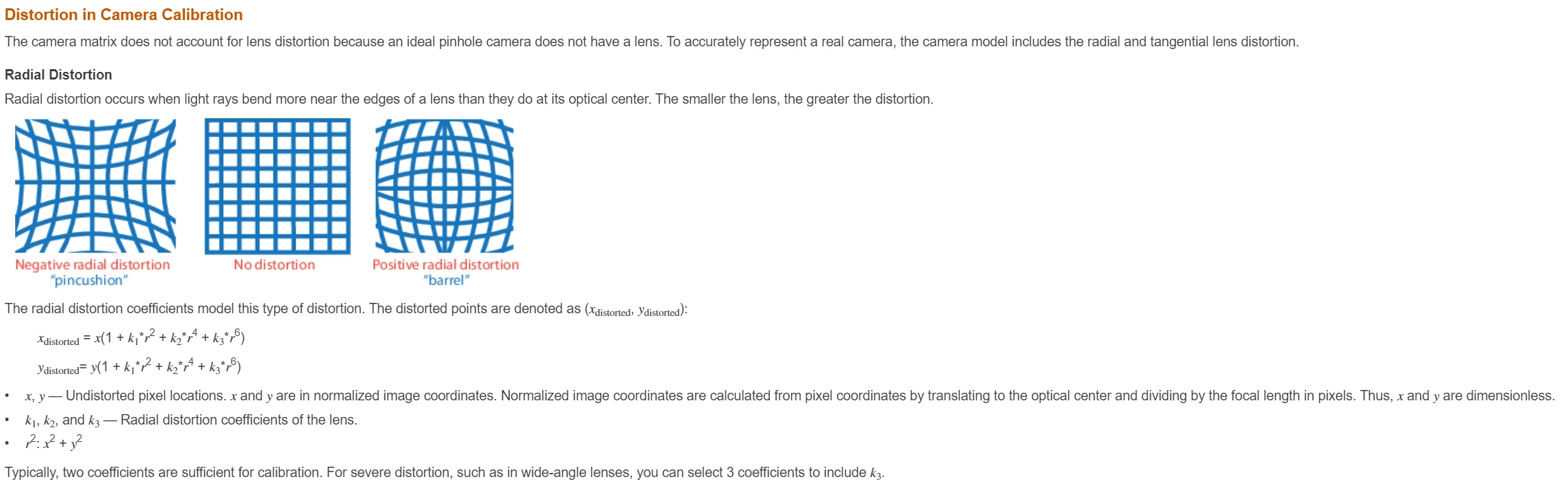Question

• 5 replies
• 794 views

• Monochrome
• 2 replies

Does anyone have an OpticStudio macro which converts the disortions written out using the DISA and DISG commands into radial distortion coefficients (k1, k2), which are typically used in camera systems?

### 5 replies

Userlevel 6+2

Hi Michael,

I've looked around and I do not see a macro that performs the actions you're looking for in our files at Zemax. I would be very interested to see if someone else has something that can perform this calculation!

In the meantime, I'm thinking you may want to use the API to perform a conversion like this rather than the ZPL. This is because you can take advantage of curve-fitting algorithms in external programs like Mathematica and Matlab through the API. Curve fitting is going to be easier to write there than in the ZPL. At this time, I'm thinking you can perform the following steps:

1. Extract the data from the Grid Distortion tool. This tool performs the same calculations as DISG and DISA, but allows you to see point-by-point data across the image. This is necessary to compare to the radial distortion coefficients which are given in terms of normalized Image coordinates.

2. Calculate the x_distorted and y_distorted value from the Grid Distortion tool for different locations across the Image

3. Normalize the image locations and set the values from step 2 equal to the equation given here: https://www.mathworks.com/help/vision/ug/camera-calibration.html

4. Use an external curve-fitting algorithm (such as FindFit in Mathematica) to calculate the coefficients from the OS data

Would something like that work for you? You can make this calculation into an extension within OpticStudio if this is a calculation you perform often! We have some guidance on creating User Extensions in the Knowledgebase article 'How to create a ZOS-API User Extension to convert from Chebyshev to Extended polynomial.'

Have a great weekend!

Best,

Allie

Userlevel 6+3

Hi Michael and Allie,

This is really interesting. It's the first time I have heard of radial distortion coefficients, so I Googled it and found https://www.pcigeomatics.com/geomatica-help/concepts/orthoengine_c/Chapter_46.html. Assuming the definition of radial distortion there is what Michael wants:

`delta r = R0 + R1*r + R2*r2 + R3*r3 + R4*r4 + R5*r5 + R6*r6+ R7*r7`

`where:`

`r: radial distance from the center of the image`

`R0 to R7: radial distortion coefficients`

then what OS produces isand OS could easily do the curve fitting needed to give these coefficients. Even better, you could write an operand that controls the coefficients directly in optimization, and by differentiating this equation you can get the maximum distortion at whatever field point it occurs at directly. It's usually at the edge of field but not always.

I think it's worth passing this to Akil for consideration of inclusion in a forthcoming release.

Userlevel 6+2

Hi Mark,

Thanks for the insight! I have created the feature request for this. I will let you and Michael know the status when the Product team responds. I'm curious to see if anyone else has a macro or extension for a calculation like this. Michael - would you say these coefficients are an industry standard? Like Mark, I haven't had to use them before. If they are something frequently cited, I will be sure to increase the priority of the feature request.

Best,

Allie

Hi Mark and Allie,

Thanks so much for looking into this for me.  This is actually the first I've been asked to provide radial distortion coefficients as well.  An engineer from the camera team has requested this information, which is what prompted me to look into it.  I believe they are using these coefficients as a spec in working with a camera lens manufacturer, so this is what to quantify the distortion we're expecting.  If this could be rolled into a new feature, that would be outstanding.  In the meantime I can look into Allie's suggestion of getting Matlab or Mathematica involved in the curve fitting.

Thanks again to you both!

Mike